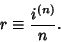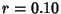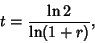## Compound Interest

Letbe the Principal (initial investment),be the annual compounded rate,the nominal rate,''be the number of times Interest is compounded per year (i.e., the year is divided intoConversion Periods), andbe the number of years (the term''). The Interest rate per Conversion Period is then(1)

If interest is compoundedtimes at an annual rate of(where, for example, 10% corresponds to), then the effective rate overthe time (what an investor would earn if he did not redeposit his interest after each compounding) is(2)

The total amount of holdingsafter a timewhen interest is re-invested is then(3)

Note that even if interest is compounded continuously, the return is still finite since(4)

where e is the base of the Natural Logarithm.

The time required for a given Principal to double (assumingConversion Period) is given by solving(5)

or(6)

where Ln is the Natural Logarithm. This function can be approximated by the so-called Rule of 72:(7)

See also e, Interest, Ln, Natural Logarithm, Principal, Rule of 72, Simple Interest

References

Kellison, S. G. The Theory of Interest, 2nd ed. Burr Ridge, IL: Richard D. Irwin, pp. 14-16, 1991.

Milanfar, P. A Persian Folk Method of Figuring Interest.'' Math. Mag. 69, 376, 1996.